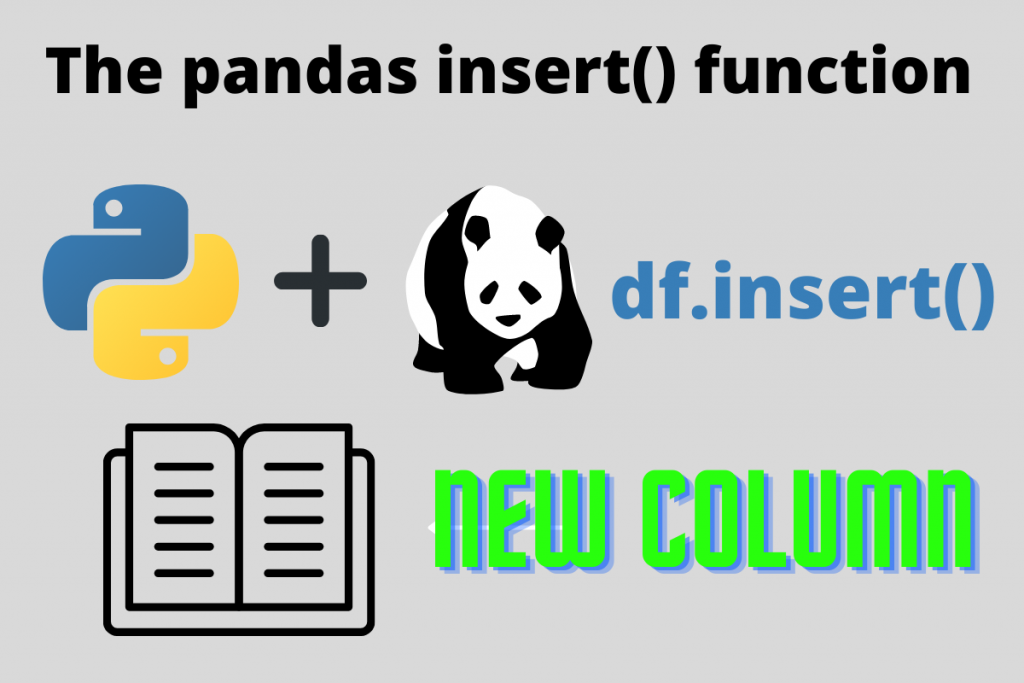# The Pandas dataframe.insert() function – A Complete GuideIn this article, we will see the dataframe.insert() function from Pandas. This function is in use for the column transformation techniques. So, let us jump right into it!

Pandas library is one of the most important libraries that collects the data and represents it for the user. This API is built upon the matplotlib and NumPy libraries which depicts that it is purely Python-made. From reading complex and huge datasets to implementing statistical analysis to them this package is very simple to learn and use.

### What is a dataframe?

The concept of a dataframe is not new to Pandas users. It’s a collection of rows and columns representing data in a tabular format. Just like a normal but we can modify each cell of the table using some lines of code. This saves a lot of work time as we do not need to search for that element in the whole table.

We will create a sample dataset and then move towards further implementation. To create one it has to be given a dictionary that has columns in the form of keys and rows in the form of values.

Code:

```import pandas as pd

data_dict = {'Name':['Ramesh', 'Suresh', 'Rajeev', 'Neha', 'Aditi'],
'Roll_nos':[1, 2, 3, 4, 5],
'Division':['A', 'B', 'C', 'D', 'E'] }

data = pd.DataFrame(data_dict)
data
```

Output:

Now that we are ready with our basic dataset let us retrieve some info about it.

### Accessing basic information from Pandas dataframes

Code:

```data.columns
data.info()
```

Output images:

### Using the dataframe.insert() function to add a column

We head towards the insertion of columns in our dataframe. The insert function is for explicitly placing any column with their values. The documentation of this is as follows:

```Signature: data.insert(loc, column, value, allow_duplicates=False) -> None
Docstring:
Insert column into DataFrame at specified location.

Raises a ValueError if `column` is already contained in the DataFrame,
unless `allow_duplicates` is set to True.

Parameters
----------
loc : int
Insertion index. Must verify 0 <= loc <= len(columns).
column : str, number, or hashable object
Label of the inserted column.
value : int, Series, or array-like
allow_duplicates : bool, optional
File:      c:\users\lenovo\anaconda3\lib\site-packages\pandas\core\frame.py
Type:      method
```

This function has a very simple syntax:

```data.insert(loc, column, value, allow_duplicates = False)
```

Explanation:

1. loc = the index location of the dataframe where we want to insert the column.
2. column = name of our column we want to insert
3. value = all the values that the column beholds
4. allow_duplicates = this attribute is for the placing the duplicate columns in hte dataframe

## Methods to use dataframe.insert()

There are two methods to use this function.

1. Directly calling the function and giving all the parameters to it.
2. Using a functional approach (recommended)

### 1. Directly calling the dataframe.insert() function

In this example, we will add a column in our dataframe by directly calling it. It is a student database so, we will try to add the Marks columns in this.

Code:

```mrk_list = [90.00, 60.06, 72.32, 78.9, 83.9] # creating a list of values for the Marks column
data.insert(3, 'Marks', mrk_list) # using the isnert function
data # calling the dataframe
```

Output:

Explanation:

1. We create a list called ‘mrk_list‘ of all values for our new “Marks” column. It will be for the values parameter.
2. Then we call the insert() function using the dot – “.” operator with our dataframe. Then we assign the location of our new column in the third index.
3. We give the name of the column. Then we assign the value as mrk_list. In this way, we add student marks to the dataset.
4. Note: When passing the values in the form of a list, make sure you do not exceed above the index values of the main dataframe

### Code for overall understanding

```# importing the module
import pandas as pd

# creating a sample dataframe
data_dict = {'Name':['Ramesh', 'Suresh', 'Rajeev', 'Neha', 'Aditi'],
'Roll_nos':[1, 2, 3, 4, 5],
'Division':['A', 'B', 'C', 'D', 'E'] }

# storing it in a variable
data = pd.DataFrame(data_dict)

# retrieving the basic info
data.info()
data.describe()

# using the insert function
mrk_list = [90.00, 60.06, 72.32, 78.9, 83.9]
data.insert(3, 'Marks', mrk_list)
data
```

### 3. Using an explicit function for a better approach

We can call that function according to our need just changing the parameters inside it. It will hold four parameters.

1. Dataframe
2. Column name
3. Location index
4. Values

Code:

```def create_column(df = None, col_name = '', col_loc = 0, values = []):
print('Creating and adding a new column')
df.insert(col_loc, col_name, values)

return df.head() # returning the new dataset with the new columns

df = data # dataframe name
col_name = input('Enter the column name: ').strip()
col_loc = int(input('Ente the location where you want to insert the column: '))
values = ['March', 'April', 'June', 'May', 'Februray']

# calling the function with the values
create_column(df, col_name, col_loc, values)

```

Output:

Explanation:

1. First we create a function as create_column().
2. It takes four parameters as
1. dataframe
2. column name
3. column location
4. values – an array of input values for the column